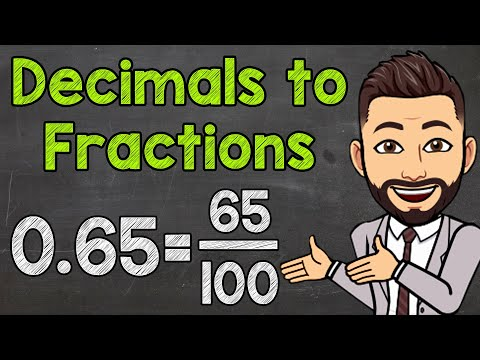Home » What is 3.875 as a Fraction? – Decimal to Fraction Conversion

# What is 3.875 as a Fraction? – Decimal to Fraction Conversion

What is 3.875 as a Fraction?

• A. 31/8
• B. 2/1
• C. 1/2
• D. 48/5
• E. 129/100

## Solution:

You can write the number 3.875 as the fraction 31/8.

Here’s how it can be converted:

The whole number is the number to the left of the decimal point. So the whole number is 3.

The fractional part is the number to the right of the decimal, 0.875. Since there are three numbers after the decimal point, you may convert this into a fraction by thinking of it as 875/1000.

Make the fraction 875/1000 as simple as possible. Since both numbers are less than 125, you get 7/8.

3 7/8 is the sum of the whole number and the fraction part.

Finally, turn the mixed fraction into the wrong fraction. The number 8 stays the same in the denominator. Multiply the whole number by the denominator and add the numerator of the fractional part to get the numerator: 3*8 + 7 = 31. So, 31/8 is the fraction.

So, 3.875 as a fraction is 31/8.

## Identifying the Whole Number and the Decimal

The 3.875 in its decimal form is made up of a whole integer and a decimal. Left of the decimal point, the complete number reads 3. There are 0.875 digits after the decimal point. These two components must be isolated before you start the conversion.

## Converting the Decimal into a Fraction

The 0.875 in the decimal place can be written as a fraction. Three digits after the decimal point indicate the thousandth place. Therefore, 0.875 has that value. Thus, 875/1000 is another way to write the fraction 0.875. There is a simpler way to write this fraction, though. It is possible to divide both the numerator and denominator by 125. The resulting fraction is 7/8.

## Combining the Whole Number and the Fraction

We now have two parts: the whole number 3 and the fraction 7/8. When you add these together, you get the mixed number 3 7/8. If you want to write the number as a single fraction, change it to an incorrect fraction. The 8 stays in the denominator. For the numerator, you multiply the whole number (3) by the denominator (8) and then add the numerator of the fraction (7). This gives 3*8 + 7 = 31. So, 3.875 as a single fraction is 31/8.

So,  3.875 is equivalent to the fraction 31/8.